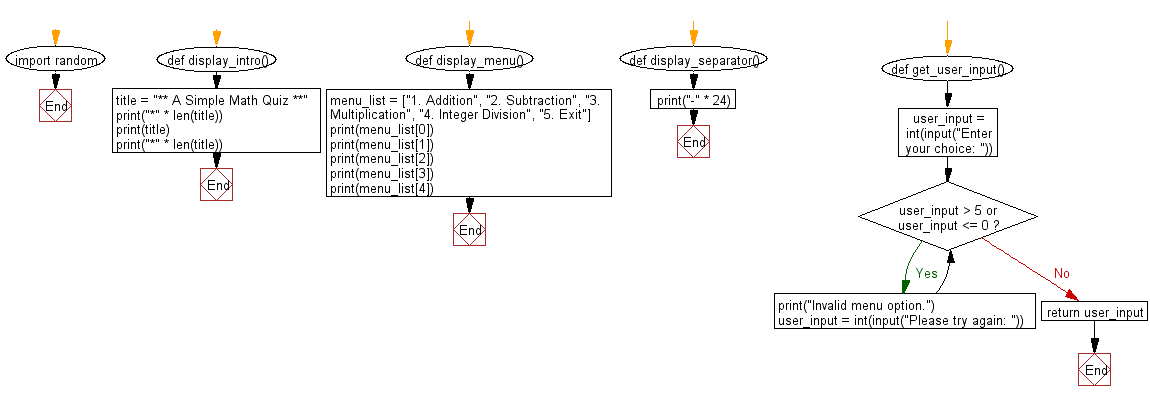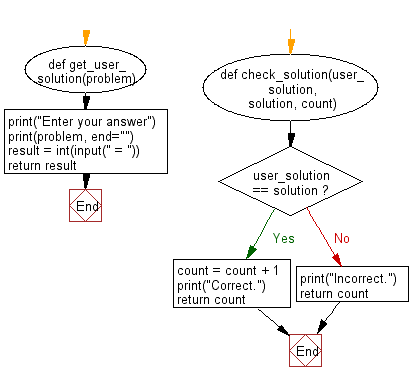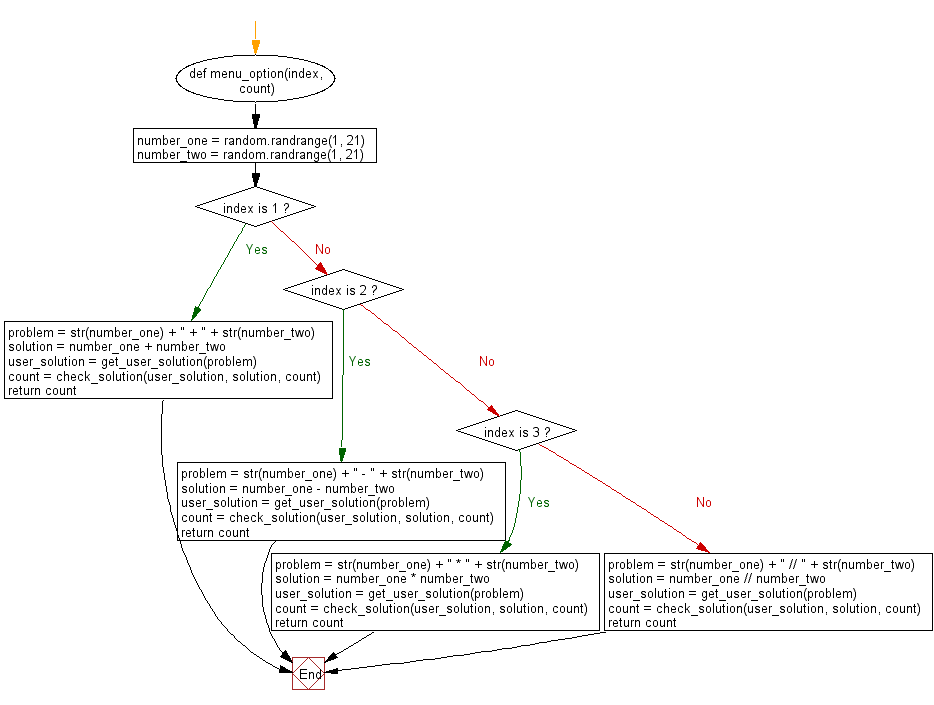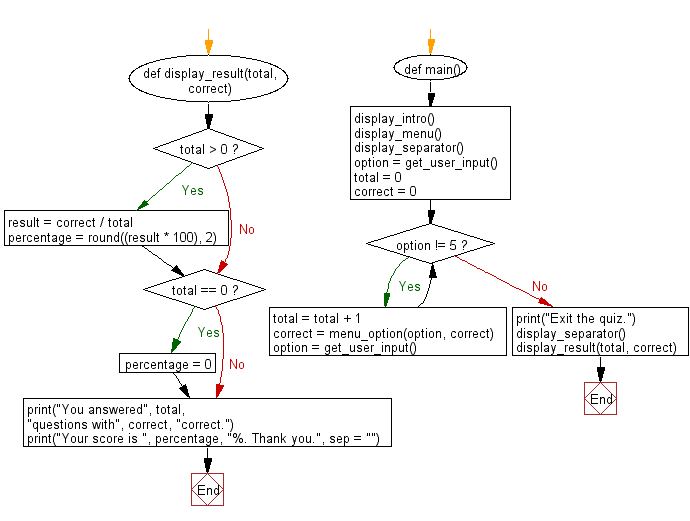﻿ Python Math: Create a simple math quiz - w3resource# Python Math: Create a simple math quiz

## Python Math: Exercise-63 with Solution

Write a Python program to create a simple math quiz.

Sample Solution:-

Python Code:

``````import random

def display_intro():
title = "** A Simple Math Quiz **"
print("*" * len(title))
print(title)
print("*" * len(title))

menu_list = ["1. Addition", "2. Subtraction", "3. Multiplication", "4. Integer Division", "5. Exit"]

def display_separator():
print("-" * 24)

def get_user_input():
user_input = int(input("Enter your choice: "))
while user_input > 5 or user_input <= 0:
user_input = int(input("Please try again: "))
else:
return user_input

def get_user_solution(problem):
print(problem, end="")
result = int(input(" = "))
return result

def check_solution(user_solution, solution, count):
if user_solution == solution:
count = count + 1
print("Correct.")
return count
else:
print("Incorrect.")
return count

number_one = random.randrange(1, 21)
number_two = random.randrange(1, 21)
if index is 1:
problem = str(number_one) + " + " + str(number_two)
solution = number_one + number_two
user_solution = get_user_solution(problem)
count = check_solution(user_solution, solution, count)
return count
elif index is 2:
problem = str(number_one) + " - " + str(number_two)
solution = number_one - number_two
user_solution = get_user_solution(problem)
count = check_solution(user_solution, solution, count)
return count
elif index is 3:
problem = str(number_one) + " * " + str(number_two)
solution = number_one * number_two
user_solution = get_user_solution(problem)
count = check_solution(user_solution, solution, count)
return count
else:
problem = str(number_one) + " // " + str(number_two)
solution = number_one // number_two
user_solution = get_user_solution(problem)
count = check_solution(user_solution, solution, count)
return count

def display_result(total, correct):
if total > 0:
result = correct / total
percentage = round((result * 100), 2)
if total == 0:
percentage = 0
print("You answered", total, "questions with", correct, "correct.")
print("Your score is ", percentage, "%. Thank you.", sep = "")

def main():
display_intro()
display_separator()

option = get_user_input()
total = 0
correct = 0
while option != 5:
total = total + 1
option = get_user_input()

print("Exit the quiz.")
display_separator()
display_result(total, correct)

main()
```
```

Sample Output:

```************************
** A Simple Math Quiz **
************************
2. Subtraction
3. Multiplication
4. Integer Division
5. Exit
------------------------
16 + 16 = 32
Correct.
Exit the quiz.
------------------------
You answered 1 questions with 1 correct.
Your score is 100.0%. Thank you.
```

Flowchart:## Visualize Python code execution:

The following tool visualize what the computer is doing step-by-step as it executes the said program:

Python Code Editor:

Have another way to solve this solution? Contribute your code (and comments) through Disqus.

What is the difficulty level of this exercise?

Test your Programming skills with w3resource's quiz.

﻿

```>>> students = [{'name': 'John', 'score': 98}, {'name': 'Mike', 'score': 94}, {'name': 'Jennifer', 'score': 99}]